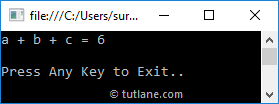In visual basic, Method Overloading means defining multiple methods with the same name but with different parameters. By using Method Overloading, we can perform different tasks with the same method name by passing different parameters.

Suppose, if we want to overload a method in visual basic, we need to define another method with the same name but with different signatures. In visual basic, the Method Overloading is also called as compile time polymorphism or early binding.

Following is the code snippet of implementing a method overloading in a visual basic programming language.

Public Class Calculate

Public Sub AddNumbers(ByVal a As Integer, ByVal b As Integer)

Console.WriteLine("a + b = {0}", a + b)

End Sub

Public Sub AddNumbers(ByVal a As Integer, ByVal b As Integer, ByVal c As Integer)

Console.WriteLine("a + b + c = {0}", a + b + c)

End Sub

End Class

If you observe the above “Calculate” class, we defined two methods with the same name (AddNumbers) but with different input parameters to achieve method overloading in visual basic.

Following is the example of implementing a method overloading in a visual basic programming language.

Module Module1

Public Class Calculate

Public Sub AddNumbers(ByVal a As Integer, ByVal b As Integer)

Console.WriteLine("a + b = {0}", a + b)

End Sub

Public Sub AddNumbers(ByVal a As Integer, ByVal b As Integer, ByVal c As Integer)

Console.WriteLine("a + b + c = {0}", a + b + c)

End Sub

End Class

Sub Main(ByVal args As String())

Dim c As Calculate = New Calculate()

Console.WriteLine("Press Enter Key to Exit..")

End Sub

End Module

When we execute the above visual basic program, we will get the result as shown below.This is how we can implement method overloading in visual basic by defining multiple methods with the same name but with different signatures based on our requirements.